# Diac

## Diac

A diac is a two terminal, three layer bidirectional device which can be switched from its OFF state to ON state for either polarity of applied voltage.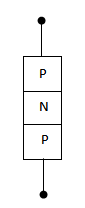Fig.1(i)

The diac can be constructed in either npn or pnp form.

Fig.1(i) shows the basic structure of a diac in pnp form. The two leads are connected to p-regions of silicon separated by an n-region.

The structure of diac is very much similar to that of a transistor. However, there are several important differences:

1. There is no terminal attached to the base layer.
2. The three regions are nearly identical in size.
3. The doping concentrations are identical to give the device symmetrical properties.

Fig.1 (ii) shows the symbol of a diac.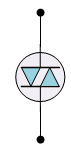Fig.1(ii)

### Operation of Diac

When a positive or negative voltage is applied across the terminal of a diac, only a small leakage current IBO will flow through the device.

As the applied voltage is increased, the leakage current will continue to flow until the voltage reaches the breakover voltage VBO.

At this point, avalanche breakdown of the reverse biased junction occurs and the device exhibits negative resistance i.e. current through the device increases with decreasing values of applied voltage.

The voltage across the device then drops to breakback voltage VW.

Fig.2 shows the V-I characteristics of a diac.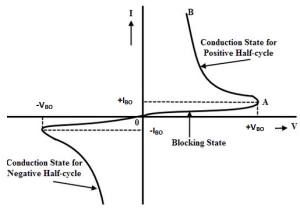Fig.2

For applied positive voltage less than +VBO and negative voltage less than –VBO , a small leakage current (±IBO) flows through the device.

Under such condition, the diac blocks the flow of current and effectively behaves as an open circuit.

The voltage +VBO and –VBO  are the breakdown voltages and usually have a range of 30 to 50 Volts.

When the positive or negative applied voltage is equal to or greater than the breakdown voltage, diac begins to conduct and the voltage drop across it becomes a few volts.

Conduction then continues until the device current drops below its holding current.

Note that the breakover voltage and holding current values are identical for the forward and reverse regions of operation.

Diacs are used primarily for triggering of triacs in adjustable phase control of a.c. mains power. Some of the circuit applications of diac are

1. light dimming
2. heat control
3. universal motor speed control

### Applications of Diac

1. #### Lamp Dimmer

Fig.3 shows a typical circuit that may be used for smooth control of a.c. power fed to a lamp.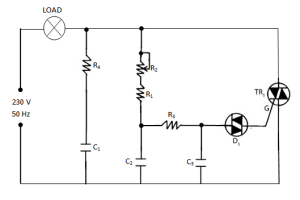Fig.3

This permits to control the light output from he lamp. The basic control is by an RC variable gate voltage arrangement.

The series R4-C1 circuit across the triac is designed to limit the rate of voltage rise across the device during switch off.

The circuit action is as follows:

As the input voltage increases positively or negatively, C1 and C2 charge at a rate determined primarily by R2.

When the voltage across C3 exceeds the breakover voltage of the diac, the diac is fired into the conducting state.

The capacitor C3 discharges through the conducting diac into the gate of the triac.

Hence, the triac is turned on to pass the a.c. power to the lamp.

By adjusting the value of R2, the rate of change of capacitors and hance the point at which triac will trigger on the positive or negative half-cycle of input voltage can be controlled.

Fig.4 shows the waveforms of supply voltage and load voltage in the diac-triac control circuit.

(i)   Supply Voltage                                                                    (ii)   Load Voltage

Fig.4

The firing of triac can be controlled upto a maximum of 180o. In this way, we can provide a continuous control of load voltage from practically zero to full r.m.s. value.

#### 2.  Heat control

Fig.5 shows a typical diac-triac circuit that may be used for the smooth control of a.c. power in a heater.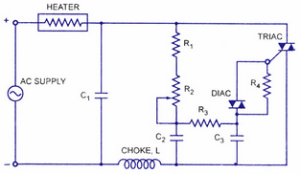Fig.5

This is similar to the circuit shown in fig.3. The capacitor C1  in series with choke L across the triac helps to slow-up the voltage rise across the device during switch-off.

The resistor R4 in parallel with the diac ensures smooth control at all positions of variable resistance R2.

### Circuit Action of Diac

As the input voltage increases positively or negatively, C1 and C2 charge at a rate determined primarily by R2.

When the voltage across C3 exceeds the breakover voltage of the diac, the diac conducts.

The capacitor C3 discharges through the conducting diac into the gate of the triac.

This turns on the triac and hence a.c. power to the heater.

By adjusting the value of R2, any portion of positive and negative half-cycles of the supply voltage can be passed through the heater.

This permits a smooth control of the heat output from the heater.